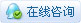# [IDE系列教程] 常用的模块 array 3.1.4

#### 类

class array.array(typecode[, iterable]）

``````typecode：数组的类型
iterable：数组初始内容``````

``````>>> import array
>>> a = array.array('i', [2, 4, 1, 5])
>>> b = array.array('f')
>>> print(a)
array('i', [2, 4, 1, 5])
>>> print(b)
array('f')``````

#### 类函数

##### 1. array.array.append(val)

``````>>> a = array.array('f', [3, 6])
>>> print(a)
array('f', [3.0, 6.0])
>>> a.append(7.0)
>>> print(a)
array('f', [3.0, 6.0, 7.0])``````
##### 2. array.array.extend(iterable)

``````>>> a = array.array('i', [1, 2, 3])
>>> b = array.array('i', [4, 5])
>>> a.extend(b)
>>> print(a)
array('i', [1, 2, 3, 4, 5])``````

### 相关帖子

 您需要登录后才可以回帖 登录 | 立即注册 本版积分规则 回帖并转播 回帖后跳转到最后一页